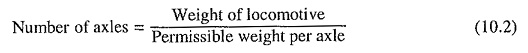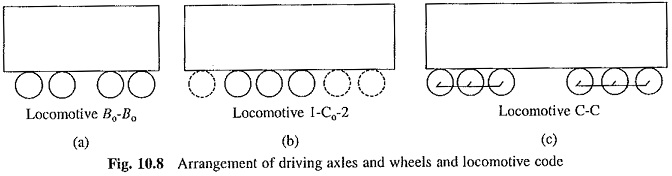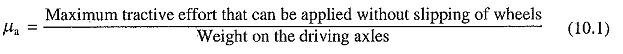## Driving Axle Code for Locomotives:

Driving Axle Code for Locomotives – Weight of the locomotive is supported on axles which are coupled to wheels. The weight per axle is limited by the strength of the track and bridges, and usually varies between 15 and 30 tons. Total number of axles is calculated by the following equation:Certain amount of torque is needed for driving a given train. The number of Driving Axle Code for Locomotives can be calculated from Eq. (10.1).

The number of Driving Axle Code for Locomotives and coupled motors are described using a code. If a locomotive possesses two driving axles, it belongs to category B. Similarly, for a 3-, 4- and 6-axle drive, the symbols used are C, B-B and C-C respectively. If each axle is driven by an individual motor; a subscript ‘o’ is used along with these symbols. In case axles are divided into groups and each group is driven by a single motor, only letters B and C are appropriately used. The number of dummy (non-driving) axles is denoted by numerals. Use of these codes is illustrated in Fig. 10.8 where dotted circles represent dummy wheels, code Bo – Bo (Fig. 10.8(a)) denotes that the locomotive has four driving axles and each axle is driven by its own driving motor. Code 1-Co-2 in Fig. 10.8(b) shows that it has three driving axles each driven by a separate motor, and 3 dummy axles. Fig. 10.8(c) indicates that the locomotive has 3 + 3 driving axles and a group of three axles is provided with one driving motor.### Difference in Construction of Main Line and Suburban Trains:In main line trains, distance between the consecutive stations is generally large. Acceleration and deceleration times form only a very small proportion of the total time of travel between the two stations. Therefore, average speed mainly depends on the free running speed and acceleration is allowed to be low to suit the passengers’ convenience. In the main line trains which are driven by locomotives, the maximum weight on driving wheels can be at most equal to the weight of locomotive. According to Eq. (10.1), for a given value of coefficient of adhesion, the torque that can be applied without wheel slip is directly proportional to weight on the driving wheels. As the weight on driving wheels is restricted to the weight of locomotive, the main line trains can have only moderate acceleration and deceleration.

In case of suburban trains, as already stated, distance between consecutive stations is usually very small. The acceleration and deceleration times form a major proportion of the total travelling time. To get a high average speed, it is necessary to reduce acceleration and deceleration times. For a given value of coefficient of adhesion, acceleration and deceleration can be increased only by increasing the ratio of the weight on driving wheels and total weight of the train. Hence, instead of a locomotive, motorized coaches are used. Each coach has its own driving motors. The usual pattern is to use the motorized and trailer coaches in the ratio of I : 2; the ratio is increased up to 1 : 1 in case of high speed trains. This arrangement allows a much higher proportion of the train weight to come on the driving wheels, compared to a locomotive, and hence, much faster acceleration and deceleration can be obtained.

Scroll to Top